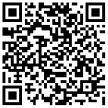18323407975首页 >> 新闻动态 >>公司新闻 >> 线切割小技巧：加工模具时间隙补偿量的确定方法
 联系电话：18323407975 邮箱：372886090@qq.com 地址：重庆九龙坡区九龙园区半山村26号

# 线切割小技巧：加工模具时间隙补偿量的确定方法

1。加工冲裁模：

f凸度=钼丝半径+单边放电间隙=0.06+0.01=0.07

f凹面=钼丝半径+单边放电间隙-配合间隙=0.06+0.01-0.015=0.055

F固体=钼丝半径+单边放电间隙+F固体=0.06+0.01+0.01=0.08

f卸荷=钼丝半径+单边放电间隙-f卸荷=0.06+0.01-0.02=0.05

2。加工冲裁模：

f凹面=钼丝半径+单边放电间隙=0.06+0.01=0.07

f凸度=钼丝半径+单边放电间隙-配合间隙=0.06+0.01-0.015=0.055

f固=钼丝半径+单边放电间隙+f固+配位间隙=0.06+0.01+0.01+0.015=0.095

f卸荷=钼丝半径+单边放电间隙+配合间隙-f卸荷=0.06+0.01+0.015-0.02=0.065

# 新闻动态

18323407975